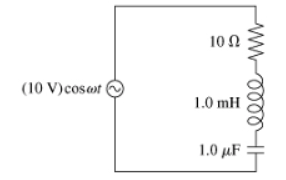# Problem: Part A. For the circuit of (Figure 1), what is the resonance frequency in rad/s?Part B. What is the resonance frequency in Hz?

###### FREE Expert Solution

Ar resonance:

$\overline{){{\mathbf{X}}}_{{\mathbf{L}}}{\mathbf{=}}{{\mathbf{X}}}_{{\mathbf{C}}}}$

XL is expressed as:

$\overline{){{\mathbf{X}}}_{{\mathbf{L}}}{\mathbf{=}}{\mathbf{\omega }}{\mathbf{L}}}$

XC is expressed as:

$\overline{){{\mathbf{X}}}_{{\mathbf{C}}}{\mathbf{=}}\frac{\mathbf{1}}{\mathbf{\omega }\mathbf{C}}}$

Part A

From the resonance equation:###### Problem DetailsPart A. For the circuit of (Figure 1), what is the resonance frequency in rad/s?

Part B. What is the resonance frequency in Hz?